## Example Questions

← Previous 1 3 4 5 6 7 8 9 60 61

### Example Question #1 : Arithmetic

Neither x nor y is equal to 0.

xy = 4y/x

Quantity A: x

Quantity B: 2

Quantity A is greater.

The relationship cannot be determined from the information given.

The two quantities are equal.

Quantity B is greater.

The relationship cannot be determined from the information given.

Explanation:

Given xy = 4y/x and x and y not 0.

Therefore you are able to divide both sides by 'y' such that:

x = 4/x

Multiply both sides by x:

x2 = 4 or x = +2 or –2.

Because of the fact that x could equal –2, the relationship cannot be determined from the information given.

### Example Question #2 : Arithmetic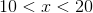Quantity A: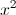Quantity B: 399

Quantitiy B is greater.

The relationship cannot be determined from the information given.

Quantity A is greater.

The two quantities are equal.

The relationship cannot be determined from the information given.

Explanation:

Sinceis between 10 and 20, it can be any real number between 100 and 400. Therefore, the relationship cannot be determined sincecould fall anywhere between these two limits, including between 399 and 400.

### Example Question #1 : How To Find The Square Of An Integer

Quantity A: 9

Quantity B: √(25 + 55)

Quantity B is greater.

Quantity A is greater.

The relationship cannot be determined from the information given.

The two quantities are equal.

Quantity A is greater.

Explanation:

In order to determine the relationship between Quantity A and Quantity B, let's convert both to square roots. In order to do this, we must square Quantity A so it becomes √81 which is equivalent to 9. Now to Quantity B, we must simplify by adding the two values together (25 + 55) to get √80.

√81 is greater than the √80 because 81 is greater than 80. Thus Quantity A is greater.

### Example Question #1 : Arithmetic

Simplify: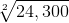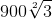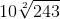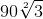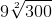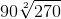Explanation:When simplifying the square root of a number that may not have a whole number root, it's helpful to approach the problem by finding common factors of the number inside the radicand. In this case, the number is 24,300.

What are the factors of 24,300?

24,300 can be factored into: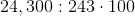When there are factors that appear twice, they may be pulled out of the radicand. For instance, 100 is a multiple of 24,300. When 100 is further factored, it is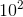(or 10x10). However, 100 wouldn't be pulled out of the radicand, but the square root of 100 because the square root of 24,300 is being taken. The 100 is part of the24,300. This means that the problem would be rewritten as:But 243 can also be factored: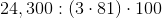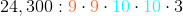Following the same principle as for the 100, the problem would become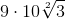because there is only one factor of 3 left in the radicand. If there were another, the radicand would be lost and it would be 9*10*3.
9 and 10 may be multiplied together, yielding the final simplified answer of### Example Question #3 : Arithmetic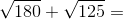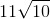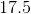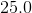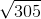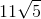Explanation:

To solve the equation, we can first factor the numbers under the square roots.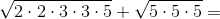When a factor appears twice, we can take it out of the square root.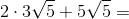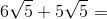Now the numbers can be added directly because the expressions under the square roots match.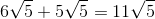### Example Question #3 : Simplifying Square Roots

Simplify.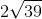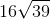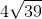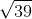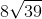Explanation:

To simplify, we must try to find factors which are perfect squares. In this case 16 is a factor of 624 and is also a perfect square.

Therefore we can rewrite the square root of 624 as: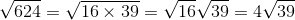### Example Question #4 : Simplifying Square Roots

Reduce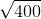to its simplest form.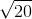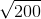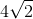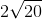Explanation:

To simplify, we must try to find factors which are perfect squares. In this case 20 is a factor of 400 and is also a perfect square.

Thus we can rewrite the problem as: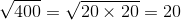Note: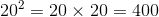### Example Question #5 : Simplifying Square Roots

Simplify.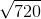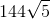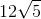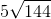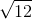Explanation:

Use the following steps to reduce this square root.

To simplify, we must try to find factors which are perfect squares. In this case 144 is a factor of 720 and is also a perfect square.

Thus we can rewrite the problem as follows.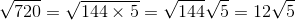### Example Question #6 : Simplifying Square Roots

Find the square root of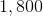.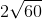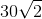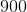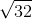Explanation:

Use the following steps to find the square root of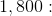To simplify, we must try to find factors which are perfect squares. In this case 900 is a factor of 1800 and is also a perfect square.

Thus we can rewrite the problem as follows.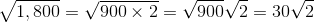### Example Question #4 : Arithmetic

Simplify.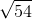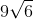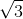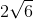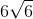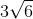Explanation:

To simplify, we must try to find factors which are perfect squares. In this case 9 is a factor of 54 and is also a perfect square.

To reduce this expression, use the following steps: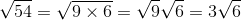← Previous 1 3 4 5 6 7 8 9 60 61

Tired of practice problems?

Try live online GRE prep today.